# Annulus

The radius of the larger circle is 8cm, the radius of smaller is 5cm. Calculate the contents of the annulus.

S =  122.5221 cm2

### Step-by-step explanation: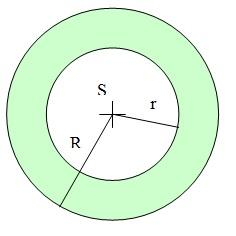Did you find an error or inaccuracy? Feel free to write us. Thank you!#### You need to know the following knowledge to solve this word math problem:

We encourage you to watch this tutorial video on this math problem:

## Related math problems and questions:

• Annulus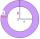Two concentric circles form an annulus of width 10 cm. The radius of the smaller circle is 20 cm. Calculate the content area of the annulus.
• Annulus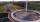Calculate the area of two circles annulus k1 (S, 3 cm) and k2 (S, 5 cm).
• Round skirt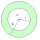The cut on the round skirt has the shape of an annulus. Determine how much m² of fabric will be consumed on an 80 cm long skirt. The circumference of the waist is a circle with a smaller radius and is 69 cm.
• Concentric circlesThere is given a Circle K with a radius r = 8 cm. How large must a radius have a smaller concentric circle that divides the circle K into two parts with the same area?
• The collarThe collar on the dress has the shape of an annulus 6 cm wide. The circumference of the inner circle is 31.4 cm. How much cm2 of fabric is needed to make one collar?
• Circle - easy 2The circle has a radius 6 cm. Calculate:
• Circle annulusThere are 2 concentric circles in the figure. Chord of larger circle 10 cm long is tangent to the smaller circle. What are does annulus have?Calculate the radius of the circle whose length is 107 cm larger than its diameterCalculate the radius of the quadrant, which area is equal to area of circle with radius r = 15 cm.
• Two annulusesThe area of the annular circle formed by two circles with a common center is 100 cm2. The radius of the outer circle is equal to twice the radius of the inner circle. Determine the outside circle radius in centimeters.
• 22/7 circleCalculate approximately the area of a circle with radius 20 cm. When calculating π, use 22/7.Find the radius of the circle with area S = 200 cm².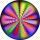Calculate the circle area with a radius of 1.2 m.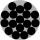Calculate the area of a circle whose radius is 5 dm.1) Calculate the circle radius if its area is 400 cm square 2) Calculate the radius of the circle whose circumference is 400 cm. 3) Calculate circle circumference if its area is 400 cm square 4) Calculate the circle's area if perimeter 400 cm.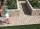Around the circular flowerbed with a radius of 2 m is a sidewalk 80 cm wide. How many square meters does the sidewalk have?How many percent of the area of a larger circle is a smaller circle if the smaller circle has a diameter 120 mm and a larger one has a diameter 300 mm?